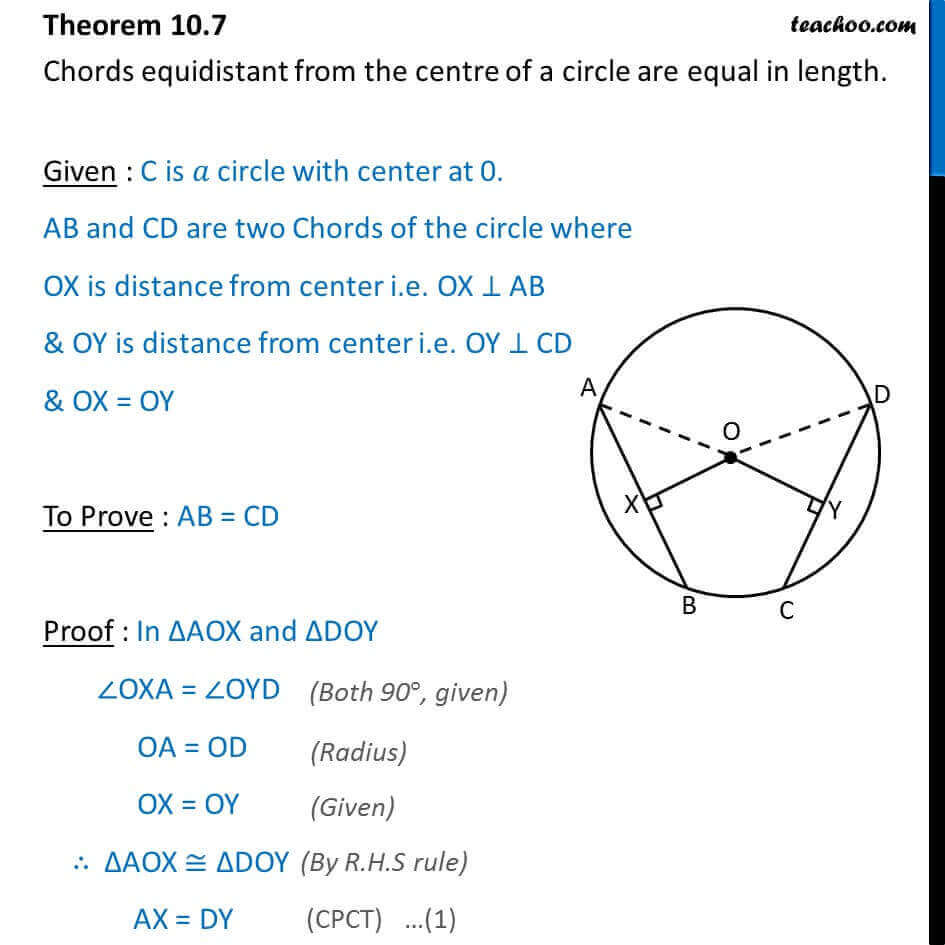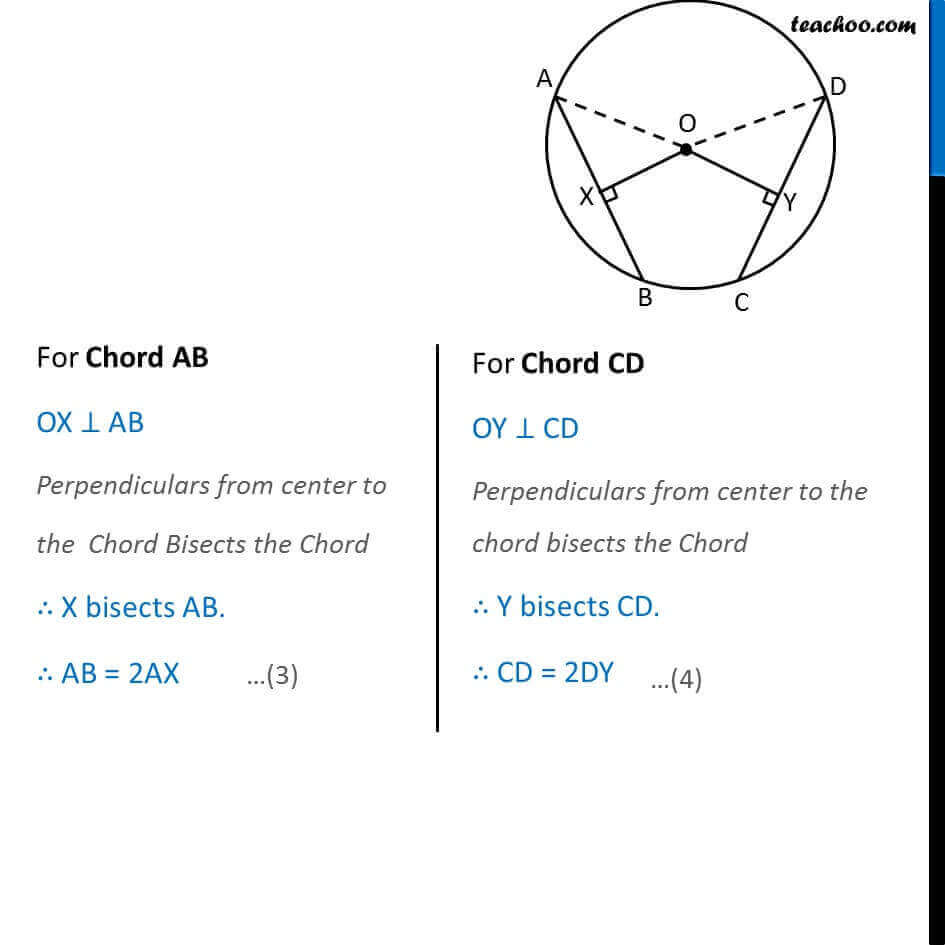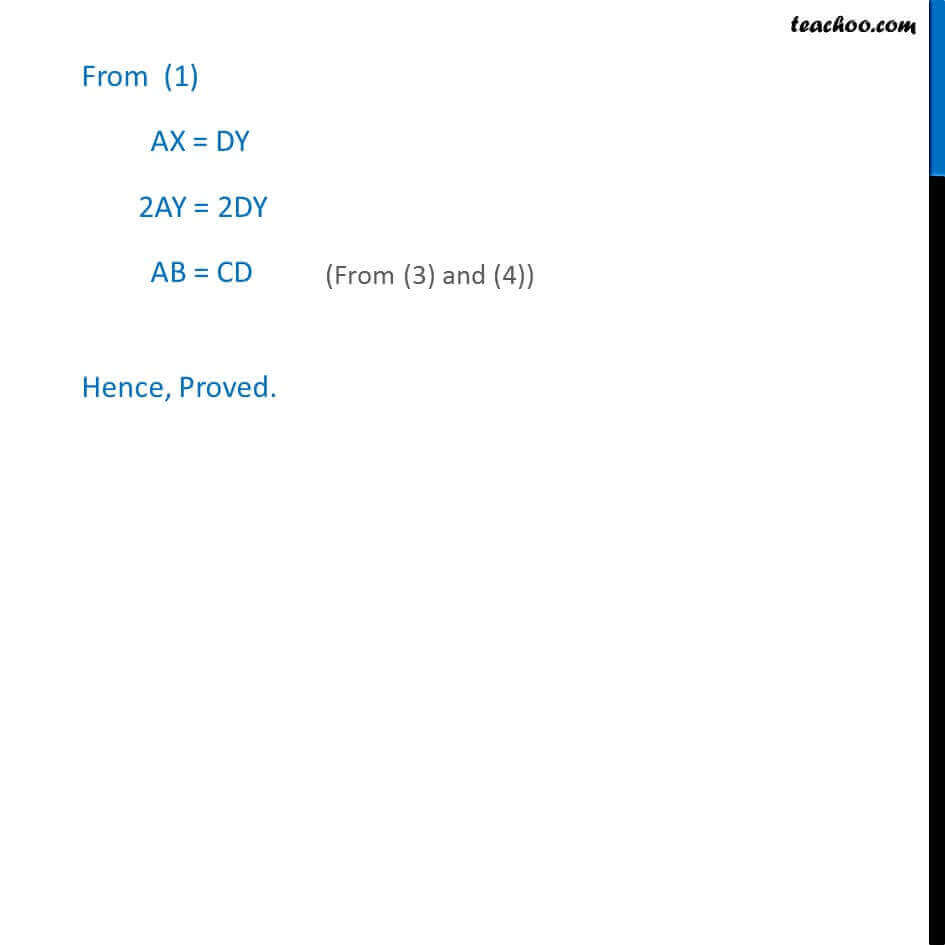Theorems

Chapter 9 Class 9 Circles
Serial order wiseLearn in your speed, with individual attention - Teachoo Maths 1-on-1 Class

### Transcript

Theorem 9.7 Chords equidistant from the centre of a circle are equal in length. Given : C is 𝑎 circle with center at 0. AB and CD are two Chords of the circle where OX is distance of chord AB from center i.e. OX ⊥ AB & OY is distance of chord AB from center i.e. OY ⊥ CD & OX = OY To Prove : AB = CD Proof : In ∆AOX and ∆CDY ∠OXA = ∠OYC OA = OC OX = OY ∴ ∆AOX ≅ ∆COY AX = CY For Chord AB OX ⊥ AB Perpendiculars from center to the Chord Bisects the Chord ∴ X bisects AB. ∴ AB = 2AX For Chord CD OY ⊥ CD Perpendiculars from center to the chord bisects the Chord ∴ Y bisects CD. ∴ CD = 2CY From (1) AX = CY 2AX = 2CY AB = CD Hence, Proved.